HOME BZU Mail Box Online Games Radio and TV Live Cricket Score All Albums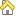BZU PAGES: Find Presentations, Reports, Student's Assignments and Daily Discussion; Bahauddin Zakariya University Multan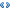Institute of ComputingBachelor of Science in Telecom SystemBsTS 1st SemesterElectrical Circuits#1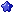bonfire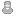M.Arsalan Qureshi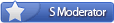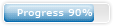Join Date: Oct 2008 Location: Garden Town, Multan Cantt Posts: 616 Program / Discipline: BSTS Class Roll Number: 09-31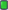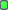Magnetic circuit

Magnetic circuit

A magnetic circuit is made up of one or more closed loop paths containing a magnetic flux. The flux is usually generated by permanent magnets or electromagnets and confined to the path by magnetic cores consisting of ferromagnetic materials like iron, although there may be air gaps or other materials in the path. Magnetic circuits are employed to efficiently channel magnetic fields in many devices such as electric motors, generators, transformers, relays, lifting electromagnets, SQUIDs, galvanometers, and magnetic recording heads.
The concept of a "magnetic circuit" exploits a one-to-one correspondence between the equations of the magnetic field in an unsaturated ferromagnetic material to that of an electrical circuit. Using this concept the magnetic fields of complex devices such as transformers can be quickly solved using the methods and techniques developed for electrical circuits.

Some examples of magnetic circuits are:
• horseshoe magnet with iron keeper (low-reluctance circuit)
• horseshoe magnet with no keeper (high-reluctance circuit)
• electric motor (variable-reluctance circuit)

Magnetomotive force (MMF)

Main article: Magnetomotive force
Similar to the way that EMF drives a current of electrical charge in electrical circuits, magnetomotive force (MMF) 'drives' magnetic flux through magnetic circuits. The term 'magnetomotive force', though, is a misnomer since it is not a force nor is anything moving. It is perhaps better to call it simply MMF. In analogy to the definition of EMF, the magnetomotive forcearound a closed loop is defined as:The MMF represents the potential that a hypothetical magnetic charge would gain by completing the loop. The magnetic flux that is driven is not a current of magnetic charge; it merely has the same relationship to MMF that electric current has to EMF. (See microscopic origins of reluctance below for a further description.)
The unit of magnetomotive force is the ampere-turn (At), represented by a steady, direct electric current of one ampere flowing in a single-turn loop of electrically conducting material in a vacuum. The gilbert (Gi), established by the IEC in 1930 , is the CGS unit of magnetomotive force and is a slightly smaller unit than the ampere-turn. The unit is named after William Gilbert (1544–1603) English physician and natural philosopher.The magnetomotive force can often be quickly calculated using Ampere's law. For example, the magnetomotive forceof long coil is:, where N is the number of turns and I is the current in the coil. In practice this equation is used for the MMF of real inductors with N being the winding number of the inducting coil.
 Magnetic flux

Main article: magnetic flux
An applied MMF 'drives' magnetic flux through the magnetic components of the system. The magnetic flux through a magnetic component is proportional to the number of magnetic field lines that pass through the cross sectional area of that component. This is the net number, i.e. the number passing through in one direction, minus the number passing through in the other direction. The direction of the magnetic field vector B is by definition from the south to the north pole of a magnet inside the magnet; outside the field lines go from north to south.
The flux through an element of area perpendicular to the direction of magnetic field is given by the product of the magnetic field and the area element. More generally, magnetic flux Φ is defined by a scalar product of the magnetic field and the area element vector. Quantitatively, the magnetic flux through a surface S is defined as the integral of the magnetic field over the area of the surfaceFor a magnetic component the area S used to calculate the magnetic flux Φ is usually chosen to be the cross-sectional area of the component.
The SI unit of magnetic flux is the weber (in derived units: volt-seconds), and the unit of magnetic field is the weber per square meter, or tesla.
 Hopkinson's law: the magnetic analogy to Ohm's law

In electronic circuits, Ohm's law is an empirical relation between the EMFapplied across an element and the current I it generates through that element. It is written as:where R is the electrical resistance of that material. Hopkinson's law is a counterpart to Ohm's law used in magnetic circuits. The law is named after the British electrical engineer, John Hopkinson. It states thatwhereis the magnetomotive force (MMF) across a magnetic element, φ is the magnetic flux through the magnetic element, andis the magnetic reluctance of that element. (It shall be shown later that this relationship is due to the empirical relationship between the H-field and the magnetic field B, B=μH, where μ is the permeability of the material.) Like Ohm's law, Hopkinson's law can be interpreted either as an empirical equation that works for some materials, or it may serve as a definition of reluctance.
 Reluctance

Main article: Reluctance
Magnetic reluctance, or magnetic resistance, is analogous to resistance in an electrical circuit (although it does not dissipate magnetic energy). In likeness to the way an electric field causes an electric current to follow the path of least resistance, a magnetic field causes magnetic flux to follow the path of least magnetic reluctance. It is a scalar, extensive quantity, akin to electrical resistance.
The total reluctance is equal to the ratio of the (MMF) in a passive magnetic circuit and the magnetic flux in this circuit. In an AC field, the reluctance is the ratio of the amplitude values for a sinusoidal MMF and magnetic flux. (see phasors)
The definition can be expressed as:whereis the reluctance in ampere-turns per weber (a unit that is equivalent to turns per henry).
Magnetic flux always forms a closed loop, as described by Maxwell's equations, but the path of the loop depends on the reluctance of the surrounding materials. It is concentrated around the path of least reluctance. Air and vacuum have high reluctance, while easily magnetized materials such as soft iron have low reluctance. The concentration of flux in low-reluctance materials forms strong temporary poles and causes mechanical forces that tend to move the materials towards regions of higher flux so it is always an attractive force(pull).
The inverse of reluctance is called permeance.Its SI derived unit is the henry (the same as the unit of inductance, although the two concepts are distinct).
 Microscopic origins of reluctance

The reluctance of a magnetically uniform magnetic circuit element can be calculated as:where
l is the length of the element in metresμ = μrμ0 is the permeability of the material (μr is the relative permeability of the material (dimensionless), and μ0 is the permeability of free space)A is the cross-sectional area of the circuit in square metres This is similar to the equation for electrical resistance in materials, with permeability being analogous to conductivity; the reciprocal of the permeability is known as magnetic reluctivity and is analogous to resistivity. Longer, thinner geometries with low permeabilities lead to higher reluctance. Low reluctance, like low resistance in electric circuits, is generally preferred.[citation needed]
 Summary of analogy between magnetic circuits and electrical circuits

The following table summarizes the mathematical analogy between electrical circuit theory and magnetic circuit theory. This is mathematical analogy and not a physical one. Objects in the same row have the same mathematical role; the physics of the two theories are very different. For example, current is the flow of electrical charge, while magnetic flux is not the flow of any quantity.

Limitations of the analogy

When using the analogy between magnetic circuits and electric circuits, the limitations of this analogy must be kept in mind. Electric and magnetic circuits are only superficially similar because of the similarity between Hopkinson's law and Ohm's law. Magnetic circuits have significant differences, which must be taken into account in their construction:
• Electric currents represent the flow of particles (electrons) and carry power, which is dissipated as heat in resistances. Magnetic fields don't represent the "flow" of anything, and no power is dissipated in reluctances.
• The current in typical electric circuits is confined to the circuit, with very little "leakage". In typical magnetic circuits not all of the magnetic field is confined to the magnetic circuit; there is significant "leakage flux" in the space outside the magnetic cores, which must be taken into account but is difficult to calculate.
• Most importantly, magnetic circuits are nonlinear; the reluctance in a magnetic circuit is not constant, as resistance is, but varies depending on the magnetic field. At high magnetic fluxes the ferromagnetic materials used for the cores of magnetic circuits saturate, limiting the magnetic flux, so above this level the reluctance increases rapidly. The reluctance also increases at low fluxes. In addition, ferromagnetic materials suffer from hysteresis so the flux in them depends not just on the instantaneous MMF but also on the past history of MMF. After the source of the magnetic flux is turned off, remanent magnetism is left in ferromagnetic circuits, creating a flux with no MMF.
 Circuit LawsMagnetic circuit

Magnetic circuits obey other laws that are similar to electrical circuit laws. For example, the total reluctanceof reluctancesin series is:This also follows from Ampère's law and is analogous to Kirchhoff's voltage law for adding resistances in series. Also, the sum of magnetic fluxesinto any node is always zero:This follows from Gauss's law and is analogous to Kirchhoff's current law for analyzing electrical circuits.
Together, the three laws above form a complete system for analysing magnetic circuits, in a manner similar to electric circuits. Comparing the two types of circuits shows that:
• The equivalent to resistance R is the reluctance Rm
• The equivalent to current I is the magnetic flux Φ
• The equivalent to voltage V is the magnetomotive Force F
Magnetic circuits can be solved for the flux in each branch by application of the magnetic equivalent of Kirchhoff's Voltage Law (KVL) for pure source/resistance circuits. Specifically, whereas KVL states that the voltage excitation applied to a loop is equal to the sum of the voltage drops (resistance times current) around the loop, the magnetic analogue states that the magnetomotive force (achieved from ampere-turn excitation) is equal to the sum of MMF drops (product of flux and reluctance) across the rest of the loop. (If there are multiple loops, the current in each branch can be solved through a matrix equation—much as a matrix solution for mesh circuit branch currents is obtained in loop analysis—after which the individual branch currents are obtained by adding and/or subtracting the constituent loop currents as indicated by the adopted sign convention and loop orientations.) Per Ampère's law, the excitation is the product of the current and the number of complete loops made and is measured in ampere-turns. Stated more generally:(Note that, per Stokes's theorem, the closed line integral of H dot dl around a contour is equal to the open surface integral of curl H dot dA across the surface bounded by the closed contour. Since, from Maxwell's equations, curl H = J, the closed line integral of H dot dl evaluates to the total current passing through the surface. This is equal to the excitation, NI, which also measures current passing through the surface, thereby verifying that the net current flow through a surface is zero ampere-turns in a closed system that conserves energy.)
More complex magnetic systems, where the flux is not confined to a simple loop, must be analysed from first principles by using Maxwell's equations.
 History

The term reluctance was coined in May 1888 by Oliver Heaviside. The notion of “magnetic resistance” was first mentioned by James Joule  and the term "magnetomotive force” (MMF) was first named by Bosanquet. The idea for a magnetic flux law, similar to Ohm's law for closed electric circuits, is attributed to H. Rowland.
 Applications

• Air gaps can be created in the cores of certain transformers to reduce the effects of saturation. This increases the reluctance of the magnetic circuit, and enables it to store more energy before core saturation. This effect is also used in the flyback transformer.
• Variation of reluctance is the principle behind the reluctance motor (or the variable reluctance generator) and the Alexanderson alternator.
• Multimedia loudspeakers are typically shielded magnetically, in order to reduce magnetic interference caused to televisions and other CRTs. The speaker magnet is covered with a material such as soft iron to minimize the stray magnetic field.
Reluctance can also be applied to:
• Reluctance motors
• Variable reluctance (magnetic) pickups
Attached Files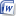Magnetic circuit (www.bzupages.com).docx (49.2 KB, 248 views)
__________________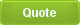Tags circuit, magneticCurrently Active Users Viewing This Thread: 1 (0 members and 1 guests)
 Thread Tools Search this Thread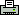Show Printable Version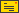Email this Page Search this Thread: Advanced Search Display Modes Rate This ThreadLinear ModeSwitch to Hybrid ModeSwitch to Threaded Mode Rate This Thread: 5 : Excellent 4 : Good 3 : Average 2 : Bad 1 : Terrible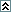Posting Rules You may not post new threads You may not post replies You may not post attachments You may not edit your posts BB code is On Smilies are On [IMG] code is On HTML code is OffTrackbacks are On Pingbacks are On Refbacks are On Forum Rules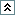Similar Threads Thread Thread Starter Forum Replies Last Post bonfire Electrical Circuits 0 17-04-2011 01:07 AM bonfire Electrical Circuits 0 17-04-2011 01:03 AM .BZU. Digital Logic and Computer Architecture 0 07-05-2009 06:45 AM .BZU. Electromagnetism 0 05-12-2008 04:16 AM .BZU. Electromagnetism 0 23-09-2008 12:26 PM

Best view in Firefox
Almuslimeen.info | Cloud Computing | Dedicated server hosting
Note: All trademarks and copyrights held by respective owners. We will take action against any copyright violation if it is proved to us.

All times are GMT +5. The time now is 02:28 AM.